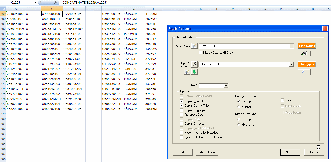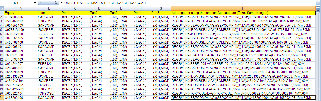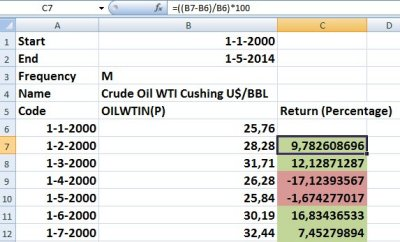## Beta and choosing the marketIn the past I have posted several times about the BETA and using the formula that is available to download a historical (rolling) BETA using Datastream. Using the formula in combination with a Request Table you can download varying BETA’s for many equities for many specific time periods.

To quickly recap, the BETA is “a measure of the risk arising from exposure to general market movements as opposed to idiosyncratic factors. The market portfolio of all investable assets has a beta of exactly 1. A beta below 1 can indicate either an investment with lower volatility than the market, or a volatile investment whose price movements are not highly correlated with the market. . . . A beta above one generally means both that the asset is volatile and tends to move up and down with the market.” (See wikipedia).

Now what can you choose as the benchmark/market if you have a mixed selection of equities from different exchanges? The benchmark is often chosen to be similar to the  chosen equities. For example, for an S&P 500 index fund and gold, the index would be the S&P 500 and the price of gold. In practice a standard index is used. If your selection of equities is large and very mixed it may not be easy to determine the standard index.

Using Datastream, however, it is possible to use the option of a static search to find out what Datastream considers to be the benchmark for each equity. It is the same static search that let’s you find out what the benchmark is when using the Datastream formula for the abnormal return using the code LI#MNEM or LI#NAME. Example:Using the codes that you then get, you can easily use Excel to generate the BETA formula that can then be used in a Request Table search for different time periods. Example:The BETA formula that I use here compares the result of 1 month to that for a period of 5 years (60 months), but the formula can be changed to days or other (sensible) options. You can download an example Excel sheet here.Email

## Return and variance of a seriesOn occasion I get asked for the return or variance of a time series. It is not always possible to download these from a database but both of them can be calculated using Excel using basic functions.

1) Return
There are many version and definitions of the return: “In finance, return is a profit on an investment. It comprises any change in value, and interest or dividends or other such cash flows which the investor receives from the investment.” Many other definitions and meanings exist and you can find an overview of some of these on Wikipedia. If I simplify it further the return means the percental change in value of a time series. As an example I have calculated the return for the price of Crude Oil WTI but the same (simplified) principle also applies to stock price series. The formula that I use is:

Return = ((Price on Day2 – Price on Day1) / Price on Day1) * 100

Below you see an example screenshot of this:2) Variance
The variance is a mathematical concept in probability theory and statistics. The variance measures how far a set of numbers is spread out. A variance of zero indicates that all the values are identical. A small variance indicates that the data tend to be very close to the mean (expected value) and hence to each other. A high variance indicates that the data are very spread out around the mean and from each other. More on variance can be found on Wikipedia as well as in many good Mathematics books.

The formula that I have used, is the one for measuring an unbiased estimate of the population variance from a fixed sample of n observations:

(s2) = S [(xi – x¯)^2] / n – 1

s2 = Variance
S = Summation, which means the sum of every term in the equation after the summation sign.
xi = Sample observation. This represents every term in the set.
= The mean. This represents the average of all the numbers in the set.
n = The sample size. You can think of this as the number of terms in the set.

Below you find an example that I created using the daily Crude Oil WTI prices from 1 January 2000 to 1 May 2014. I used a similar step by step approach as shown in WikiHow:The Excel formulas that I used were:
1) To get the sum of all the prices: =SUM(B6:B178). The reult is in cell E2.

2) To get the average I used: =AVERAGE(B6:B178). The reult is in cell D2.

3) Price (in B6 to B178) minus the average becomes: price – x¯ etc. For the values in column B it would be: B6 – \$D\$2. See the result in cells C6 – C178.

4) The square of the price minus the mean would be in column D6 – D178. Example: =(C6)^2

5) The sum of the squared prices of column D is in cell F2: =SUM(D6:D178)

6) The number of prices n is in cell G2: =COUNT(D6:D178)

7) The variance can now be calculated and is put in cell F6: =F2/(\$G\$2)-1

8) The standard deviation is the square root of the variance. I put this in cell G6: =SQRT(F6)

You can also download the example Excel-sheet here.Email